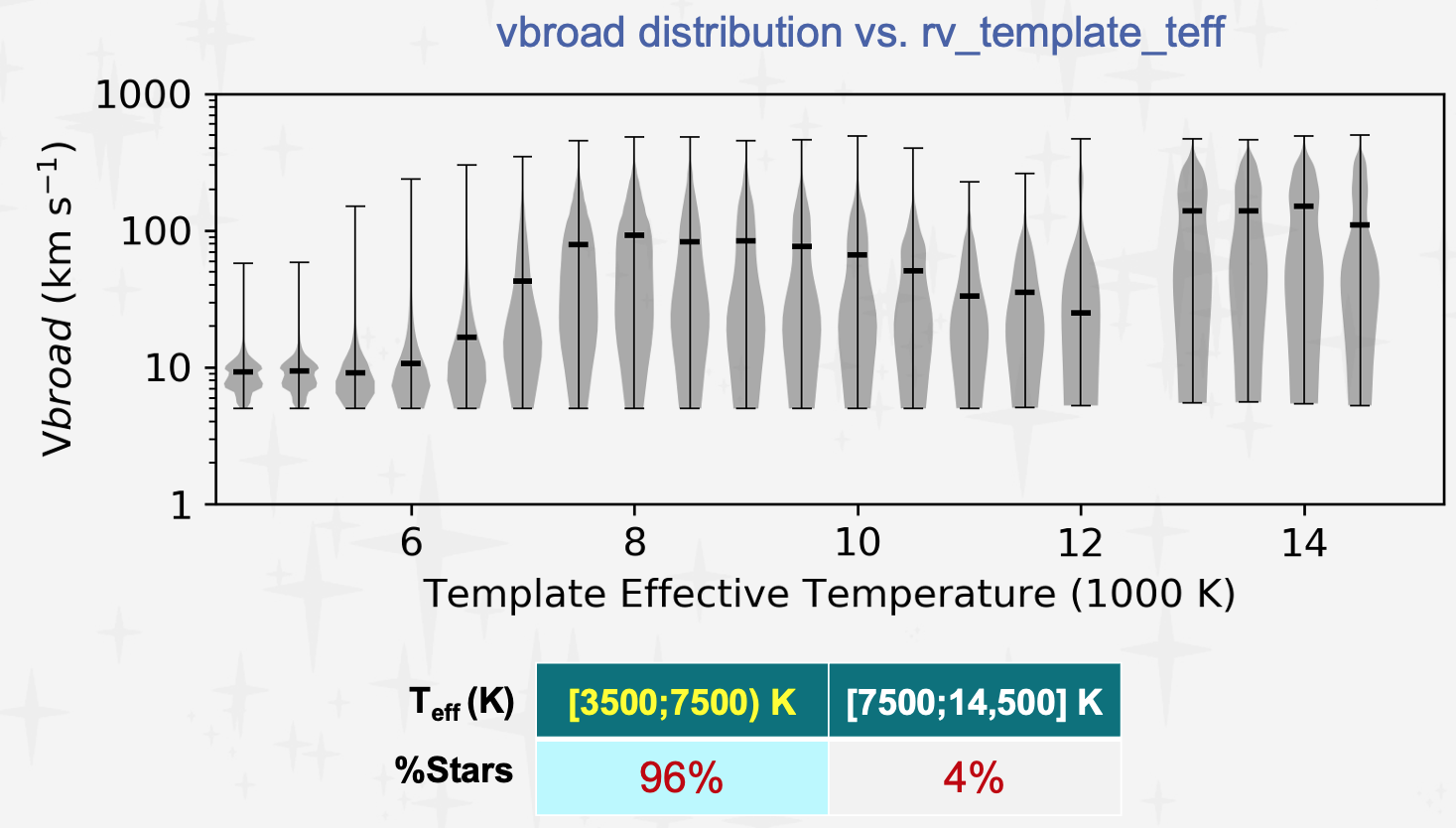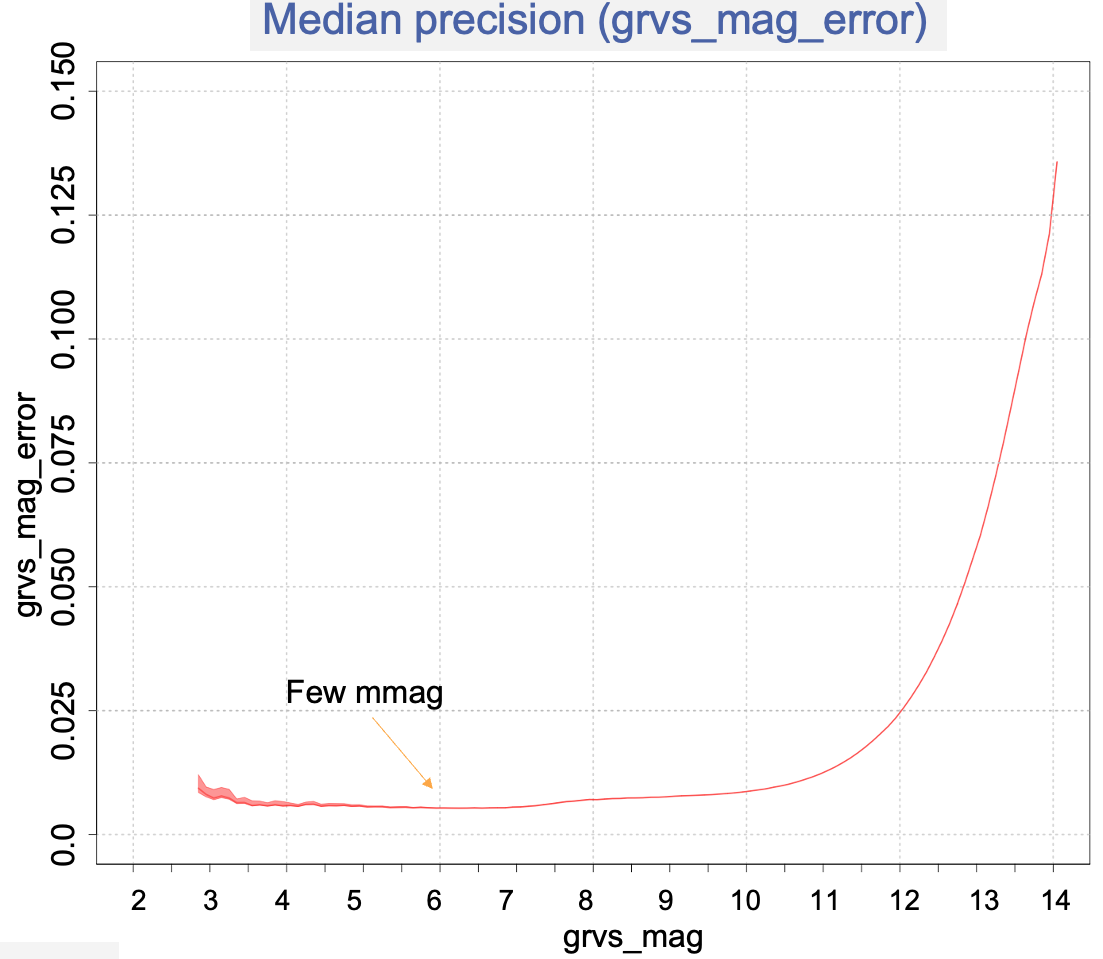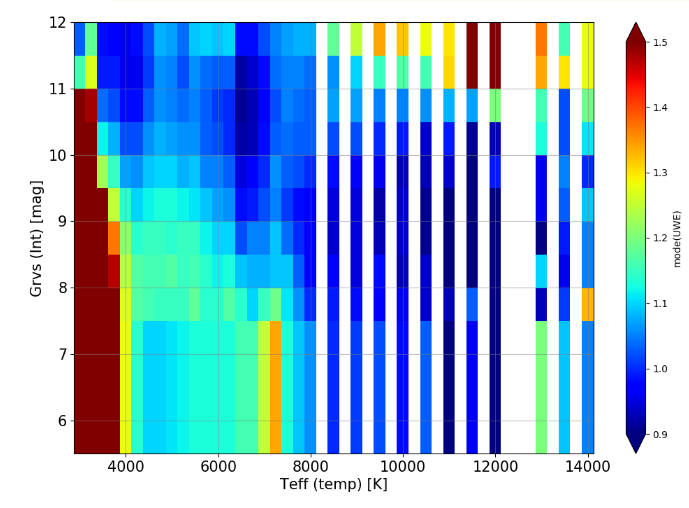# 6.5.2 Validation and Post-processing

When the CU6 Gaia DR3 processing was finished, the CU6 carried out a catalogue-level validation campaign on the pipeline products in order to assess the properties of the entire dataset and to identify the bad quality data to exclude from publication. The recipes to identify spurious data were developed in Post-processing. Then, a second catalogue-level validation campaign was carried out in collaboration with the CU9 and some additional data were filtered out.

## Filters

The main task of Post-processing is to flag as invalid the spurious radial_velocity, vbroad, grvs_mag, and rvs_mean_spectrum. The invalid data are filtered out during the ingestion in the Gaia Archive, and not published in DR3. The filters on the primary product of the RVS pipeline, the radial_velocity, are applied also to all the other products. The other products have, in addition to the radial_velocity filters, their own filters applied. The data of some more sourceids were filtered out in the second validation campaign, but the large majority were filtered in Post-processing:

• radial_velocity filters. The following radial_velocity filters are applied to all the pipeline products. A star satisfying at least one of these conditions, has its radial_velocity, vbroad, grvs_mag, and rvs_mean_spectrum removed from DR3: The total number of radial_velocity filtered out is about 4 million (3 616 153). The approximate number of sources entering each filter is also written (one source can enter in more than one filter):

• Large uncertainty: radial_velocity_error $>40$ $\rm\,km\,s^{-1}$.

• SB2 candidate: the stars having $\geq 10~{}\%$ of the transits flagged as double_lined ($\sim$ 40 000 sources).

• Hot star (Faint: grvs_mag $>12$ mag): rv_template_teff $\geq 7000$K ($\sim$ 1.7 million sources).

• Hot star (Bright: grvs_mag $\leq 12$ mag): rv_template_teff $\geq 14\,500$K; ($\sim$ 66 000 sources).

• Cool star: rv_template_teff$<3100$K ($\sim$243 000 sources).

• Emission line star candidate: the stars having $\geq 30~{}\%$ of the transits flagged as presenting emission lines.

• Bad C-function (Faint grvs_mag $>12$ mag): the combined cross correlation function is deemed spurious, based on a criterion combining the information from several parameters characterising the C-function (like the maximum value, FWHM, kurtosis, skewness, ratio and distance between the two highest peaks, the number of relatively high peaks, the signal to noise ratio). This is the most effective filter: about 2.9 million sources are filtered out.

• Large stdev (Bright: grvs_mag $\leq 12$ mag): the standard deviation of the single-transit RV measurements is $>577$ $\rm\,km\,s^{-1}$. This conservative filter was also used in DR2 and corresponds to uniform distribution between -1000; 1000 $\rm\,km\,s^{-1}$; ($\sim$ 2500 sources).

• Faint star: grvs_mag $>14.5$ mag ($\sim$ 21 000 sources).

• Too noisy spectrum: rv_expected_sig_to_noise $<2$ ($\sim$270 000 sources).

• Spurious HVS: A list of sourceId of $\sim$ 34 000 stars with spurious high velocity. This was not the complete list and the study for identifying remaining spurious HVS continued after the Post-processing run.

After the second validation campaign, an additional $\sim$ 80 000 radial_velocities have been removed from DR3. The distribution on the sky of the remaining $\sim$33.8 million of stars having radial_velocity in DR3, of their rv_template_teff, and the median precision as a function of grvs_mag are summarised in Figure 6.14. The validation and the properties of the radial_velocities published in DR3 are described in Katz et al. (2023), and, in particular, the hot star radial_velocities in Blomme et al. (2023).

• Outside range: the accepted range of vbroad is: $5\leq$ vbroad $\leq 500$ $\rm\,km\,s^{-1}$. This is the most effective filter: there are $\sim$7.7 million vbroad, before the filter, and $\sim$ 4.9 million remain.

• Too few transits: vbroad_nb_transits $\leq 5$ [remaining 4.4 million].

• Too noisy spectrum: (specSigToNoiseNoBlend) SNR $<$ 15 [remaining 4.2 million].

• Cool star: rv_template_teff $\leq$ 3500K [remove 74 000].

The distribution of the $\sim$3.5 million vbroad published in DR3 is shown in Figure 6.15, and is in reasonably good agreement with Głȩbocki and Gnaciński (2003). The description of the properties and the validation of vbroad are described in Frémat et al. (2023).Figure 6.15: This violin plot shows the distribution of vbroad as a function of rv_template_teff. The thick horizontal bars indicate the median value. About 96 % of the stars having a vbroad measurement are medium temperature stars, with 3500 K < rv_template_teff ≤ 7500 K, and 4 % are hot stars with with 7500 K ≤ rv_template_teff ≤14 500 K. The line broadening velocities vbroad published in DR3 are described in Frémat et al. (2023).
• grvs_mag filters: In addition to the radial_velocity filters mentioned above, the filters applied to grvs_mag are:

• Too few transits: grvs_mag_nb_transits $<2$ for grvs_mag $<13$ mag; grvs_mag_nb_transits $<3$ for grvs_mag $\geq 13$ mag.

• Faint star: grvs_mag $>14.1$ mag.

• Large stdev: The maximum accepted standard deviation of the epoch $G_{\rm RVS}$ measurements has been set to 3.3. As done for the radial velocities: the stdev is required to be at least smaller than what expected for a uniform distribution within [2.8; 14.1]: max(stdev)=(14.1-2.8)/sqrt(12). This filter sets invalid only about 200 grvs_mag.

After the second validation campaign an additional $\sim$ 44 000 grvs_mag were removed from DR3 because affected by contamination from nearby sources. Figure 6.16 shows the median internal precision of grvs_mag. The validation and the properties of the magnitudes grvs_mag published in DR3 are described in Sartoretti et al. (2023).Figure 6.16: The median internal precision of grvs_mag (i.e. grvs_mag_error) as a function of grvs_mag. The median is computed over bins of 0.1 magnitudes.
• rvs_mean_spectrum filters: The RVS pipeline produced one mean spectrum for each source processed (about 37 million). The brightest mean spectra are used by the atmospheric parameter pipeline (Chapter 11) to estimate the star atmospheric parameters and the abundances of some elements, and it was decided to publish about 1 million of them. A preliminary selection of the spectra candidate to publication was done by applying the following filters to the rvs_mean_spectra, in addition to the radial_velocity filters:

• rvs_spec_sig_to_noise $<$15 [remaining 9.3 million].

• nbCombinedTransits $\leq 2$ [ remove 21 000].

• Too many ($>$ 480) NaN samples [remove 3000].

• Spurious features in flux[i]: spurious emission-lines, spikes in the borders.

• Spurious features in fluxError[i].

The total number of spectra filtered in Post-processing and in the second validation campaign is $\sim$ 29 million. Among the remaining spectra, about one million was selected for publication in Gaia DR3. These include:

• 650 000 sources available for computation of Gsp-Spec astrophysical parameters with teff_gspspec between 3500 and 10 000 K

• 50 000 M stars with teff_gspphot between 3100 and 3500 K

• 50 000 B stars with teff_gspphot between 10 000 and 14 500 K

• 250 000 faint sources with low Signal-to-Noise ratios (15-25) with teff_gspphot between 3500 and 10 000K

Please notice that the sources selected for astrophysical parameters computation are not uniformly distributed due to a preference for high SNRs and the availability of data from other surveys for validation purposes. Due to a problem in the selection of the other three lists, the spatial distribution of the sources contains noticeable patterns and gaps.

The selection, the validation and the properties of the rvs_mean_spectra published in DR3 are described in Seabroke et al. (2022).

## rv_renormalised_gof

Another task of Post-processing was to estimate the radial velocity renormalised goodness of fit, rv_renormalised_gof. This is a catalogue-level task requiring the epoch radial velocities, their internal uncertainties and the median radial_velocities of all the bright stars ( grvs_mag $\leq$ 12 mag), in order to produce the Unit Weight Error (UWE) map, which is needed to compute rv_renormalised_gof (see Figure 6.17).Figure 6.17: The UWE map used to estimate rv_renormalised_gof is a function of rv_template_teff and grvs_mag. The colour scale represents the UWE statistical mode. UWE > 1 is an indication of underestimated epoch radial velocity internal uncertainties and UWE < 1 of an overestimated one. Figure by David Katz.